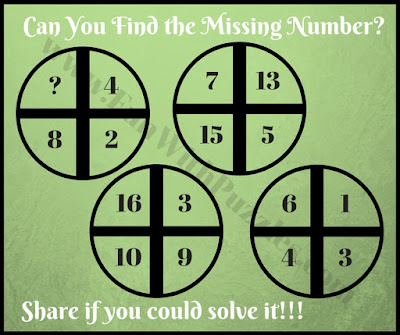Logical and Mathematical Brain Teasers are a very interesting type of puzzle. These types of brain teasers test both the Logical and Mathematical abilities of the solver. In these puzzles, one has to find out the Mathematical relationship between the given number and then solve it logically for the missing number. Circle Math Puzzles fall into this category. In these puzzles, some numbers are given in circles with are mathematical and logically related to each other. One has to find this relationship and solve it for the missing number. Today's puzzles are Cross Circle Mathematical Puzzles.

## How to Solve Picture Maths Brain Teasers

In these Cross Circle Math Logic Puzzles, the circle is divided into four segments and each segment is having four numbers. There is some Mathematical or logical connection between the given numbers. There are four such circles given in each of the puzzles. One has to study this relationship between these numbers in three circles where all four numbers are given and then find out the missing number in the fourth circle.1. Can you find the missing number in a circle quickly?2. Quick math cross-circle picture puzzle3. Mind twisting math cross-circle picture puzzle4. Thinking math cross-circle brain teaser5. Tricky math cross-circle riddle